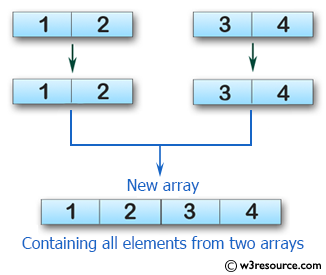﻿ Swift Array Programming Exercise: Test if an array of length four containing all their elements from two given array of integers - w3resource# Swift Array Programming Exercises: Test if an array of length four containing all their elements from two given array of integers

## Swift Array Programming: Exercise-18 with Solution

Write a Swift program to test if an array of length four containing all their elements from two given array (each length two) of integers,.

Pictorial Presentation:Sample Solution:

Swift Code:

``````func new_array(_ a: [Int], _ b: [Int]) -> [Int] {
var new_array: [Int] = []
new_array.append(contentsOf: a)
new_array.append(contentsOf: b)

return new_array
}

print(new_array([1, 2], [3, 4]))
print(new_array([5, 5], [7, 7]))
print(new_array([-1, 0], [1, 2]))
```
```

Sample Output:

```[1, 2, 3, 4]
[5, 5, 7, 7]
[-1, 0, 1, 2]
```

Swift Programming Code Editor:

Improve this sample solution and post your code through Disqus

What is the difficulty level of this exercise?

﻿